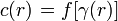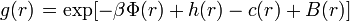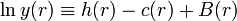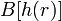2022: SklogWiki celebrates 15 years on-line

# Closure relations

When there are more unknowns than there are equations then one requires a closure relation. There are two basic types of closure schemes.

## Contents

#### Truncation Schemes

In truncation schemes, higher order moments are arbitrarily assumed to vanish, or simply prescribed in terms of lower moments. Truncation schemes can often provide quick insight into fluid systems, but always involve uncontrolled approximation.

#### Asymptotic schemes

Asymptotic schemes depend on the rigorous exploitation of some small parameter. They have the advantage of being systematic, and providing some estimate of the error involved in the closure. On the other hand, the asymptotic approach to closure is mathematically very demanding.

#### General form

The closure relation can be written in the general form$\left. c(r) \right. = f [ \gamma (r) ]$

Morita and Hiroike eased this task for a closure relation (see Ref. 1) by providing the formally exact closure formula:$\left. g(r) \right. = \exp[-\beta \Phi(r) + h(r) -c(r) +B(r)]$

or written using the cavity correlation function$\ln y(r) \equiv h(r) -c(r) +B(r)$

leaving one with the task of finding the so called bridge function$B[h(r)]$ rather than the entire closure relation.